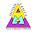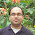## Thursday, July 19, 2018

It is the Logic Puzzle Question in Math for Teens. In this logical puzzle, you are presented with logical equation statements in the given Puzzle Picture. One has to decipher these logical equation statements to find the hidden logical pattern in these equation. Then apply the same logical pattern in the last equation to find the missing number which replaces question mark?Can you solve this Logic Puzzle Question in Math?

Answer of this "Logic Puzzle Question Math", can be viewed by clicking on button. Please do give your best try before looking at the answer.

1.1.